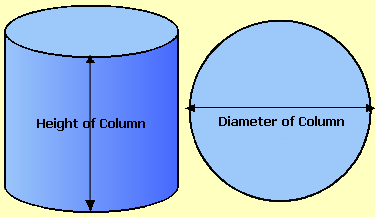Spike's Calculators

# Concrete Calculator For Columns - Metric

Calculate the amount of concrete needed for pouring columns using metric measurements.

To calculate the volume of a column (cylinder), you need to know the diameter and the height of the column.### Columns Concrete Volume - Metric

Number of Columns #
Diameter mm
Column Height m

Lineal Length of Tube m
Cubic Metres
Cubic Yards yd ³
Cubic Feet ft ³

#### Calculation

1. number of columns needed
2. column's diameter in millimetres
3. the height of the column in metres

#### Results

1. lineal metres of column forms needed
2. the amount of concrete needed in cubic metres
3. concrete needed in cubic yards
4. concrete needed in feet

##### Formula's used:
```V = Π * D² ÷ 4 * h
where V = concrete volume in cubic feet
pi = 3.14159......
d = diameter
h =  height```

##### Example calculation:
```column height = 6 metres
diameter = 300mm = 0.3 metres
needed = 5  columns
3.14159  * 0.3² ÷ 4  * 6 * 5 = 2.12 cubic metres of concrete
```

Using the formula from above;
`3.14159  * 0.3² ÷ 4  * 6 * 5 =`
`3.14159  * 0.09 ÷ 4  * 6 * 5 = 2.12 rounded to two decimals`
`You need 2.12 cubic metres of concrete for these five columns.`

##### Conversions
```To convert cubic metres to cubic yards multiply cubic metres * 1.30795061931439
To convert cubic metres to cubic feet multiply cubic metres * 35.3146667214886
```

### When Ordering Concrete

This concrete calculator will help you in estimating the amount of concrete needed for your project. The amount given as needed, does not include any waste. It is recommended, depending on the job you are doing, to add anywhere from an extra 4% to 10% to your concrete order to make sure you have enough concrete to finish the job. Ask when ordering the concrete. They will be able to give you a good idea of what is needed.